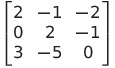Select Page

# MCQ Answers for CBSE 12 Science Maths Determinant in English

MCQ Answers for CBSE 12 Science Maths Determinant in English to enable students to get Answers in a narrative video format for the specific question.

Expert Teacher provides MCQ Answers for CBSE 12 Science Maths Determinant through Video Answers in English language. This video solution will be useful for students to understand how to write an answer in exam in order to score more marks. This teacher uses a narrative style for a question from Determinant not only to explain the proper method of answering question, but deriving right answer too.

Please find the question below and view the Answer in a narrative video format.

Question:

## Similar Questions from CBSE, 12th Science, Maths, Determinant

Question 1 :  Evaluate the determinants:. (View Answer Video)

Question 2 :  Find the values of x, if. (View Answer Video)

Question 4 :  Find the area of the triangle with vertices at the points (2, 7), (1, 1), (10, 8). (View Answer Video)

Question 5 : Let A be square matrix of order 3 * 3, then |kA|is equal to (View Answer Video)

### Matrices

Question 1 : Find the value of X, ifand. (View Answer Video)

Question 2 : If,find the value of y.  (View Answer Video)

Question 3 : If, find (x-y). (View Answer Video)

Question 4 : Find the transpose of the matrix:. (View Answer Video)

Question 5 : Compute:. (View Answer Video)

### Vector Algebra

Question 1 : Find the unit vector in the direction of the vector(View Answer Video)

Question 2 : Find the angle between the vectorsand(View Answer Video)

Question 3 : Find the magnitude of two vectors a and b having the same magnitude and such that the angle between them isand their scalar product is 1/2. (View Answer Video)

Question 4 : Find a vector in the direction of vectorwhich has magnitude 21 units.  (View Answer Video)

Question 5 : Find the scalar quantities from the following:
(i) Time period          (ii) Distance          (iii) Force
Question 3 : Ifandfindif E and F are independent events.  (View Answer Video)